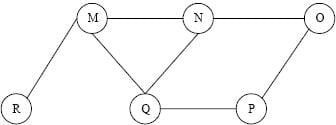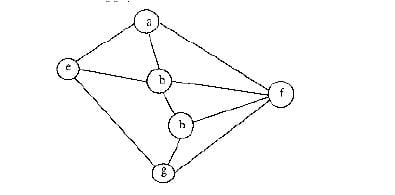Test: Graph Based Algorithms- 1

# Test: Graph Based Algorithms- 1

Test Description

## 15 Questions MCQ Test Algorithms | Test: Graph Based Algorithms- 1

Test: Graph Based Algorithms- 1 for Computer Science Engineering (CSE) 2023 is part of Algorithms preparation. The Test: Graph Based Algorithms- 1 questions and answers have been prepared according to the Computer Science Engineering (CSE) exam syllabus.The Test: Graph Based Algorithms- 1 MCQs are made for Computer Science Engineering (CSE) 2023 Exam. Find important definitions, questions, notes, meanings, examples, exercises, MCQs and online tests for Test: Graph Based Algorithms- 1 below.
Solutions of Test: Graph Based Algorithms- 1 questions in English are available as part of our Algorithms for Computer Science Engineering (CSE) & Test: Graph Based Algorithms- 1 solutions in Hindi for Algorithms course. Download more important topics, notes, lectures and mock test series for Computer Science Engineering (CSE) Exam by signing up for free. Attempt Test: Graph Based Algorithms- 1 | 15 questions in 35 minutes | Mock test for Computer Science Engineering (CSE) preparation | Free important questions MCQ to study Algorithms for Computer Science Engineering (CSE) Exam | Download free PDF with solutions
 1 Crore+ students have signed up on EduRev. Have you?
Test: Graph Based Algorithms- 1 - Question 1

### Which of the following is an advantage of adjacency list representation over adjacency matrix representation of a graph?

Detailed Solution for Test: Graph Based Algorithms- 1 - Question 1

An array of lists is used. The size of the array is equal to the number of vertices. Let the array be an array[]. An entry array[i] represents the list of vertices adjacent to the ith vertex. This representation can also be used to represent a weighted graph. The weights of edges can be represented as lists of pairs.

Test: Graph Based Algorithms- 1 - Question 2

### What are the appropriate data structures for following algorithms? 1) Breadth First Search                            2) Depth First Search                             3) Prim's Minimum Spanning Tree                  4) Kruskal' Minimum Spanning Tree

Detailed Solution for Test: Graph Based Algorithms- 1 - Question 2

1) Breadth First Search uses Queue

2) Depth First Search uses Stack

3) Prim's Minimum Spanning Tree uses Priority Queue.

4) Kruskal' Minimum Spanning Tree uses Union Find.

Test: Graph Based Algorithms- 1 - Question 3

### The degree sequence of a simple graph is the sequence of the degrees of the nodes in the graph in decreasing order. Which of the following sequences can not be the degree sequence of any graph? I. 7, 6, 5, 4, 4, 3, 2, 1 II. 6, 6, 6, 6, 3, 3, 2, 2 III. 7, 6, 6, 4, 4, 3, 2, 2 IV. 8, 7, 7, 6, 4, 2, 1, 1

Detailed Solution for Test: Graph Based Algorithms- 1 - Question 3

In sequence IV, we have a vertex with degree 8 which is not possible in a simple graph (no self loops and no multiple edges) with total vertex count as 8. Maximum possible degree in such a graph is 7.

In sequence II, four vertices are connected to 6 other vertices, but remaining 4 vertices have degrees as 3, 3, 2 and 2 which are not possible in a simple graph (no self loops and no multiple edges).

Test: Graph Based Algorithms- 1 - Question 4

The Breadth First Search algorithm has been implemented using the queue data structure. One possible order of visiting the nodes of the following graph isDetailed Solution for Test: Graph Based Algorithms- 1 - Question 4

Option (A) is MNOPQR. It cannot be a BFS as the traversal starts with M, but O is visited before N and Q. In BFS all adjacent must be visited before adjacent of adjacent. Option (B) is NQMPOR. It also cannot be BFS, because here, P is visited before O. (C) and (D) match up to QMNP. We see that M was added to the queue before N and P (because M comes before NP in QMNP). Because R is M's neighbor, it gets added to the queue before the neighbor of N and P (which is O). Thus, R is visited before O.

Test: Graph Based Algorithms- 1 - Question 5

The most efficient algorithm for finding the number of connected components in an undirected graph on n vertices and m edges has time complexity. (A) θ(n) (B) θ(m) (C) θ(m + n) (D) θ(mn)

Detailed Solution for Test: Graph Based Algorithms- 1 - Question 5

Connected components can be found in O(m + n) using Tarjan's algorithm. Once we have connected components, we can count them.

Test: Graph Based Algorithms- 1 - Question 6

Let G be an undirected graph. Consider a depth-first traversal of G, and let T be the resulting depth-first search tree. Let u be a vertex in G and let v be the first new (unvisited) vertex visited after visiting u in the traversal. Which of the following statements is always true?

Detailed Solution for Test: Graph Based Algorithms- 1 - Question 6

In DFS, if 'v' is visited
after 'u', then one of the following is true.
1) (u, v) is an edge.
u
/   \
v     w
/     / \
x     y   z

2) 'u' is a leaf node.
w
/   \
x     v
/     / \
u     y   z
In DFS, after visiting a node, we first recur for all unvisited children. If there are no unvisited children (u is leaf), then control goes back to parent and parent then visits next unvisited children.

Test: Graph Based Algorithms- 1 - Question 7

Consider an undirected unweighted graph G. Let a breadth-first traversal of G be done starting from a node r. Let d(r, u) and d(r, v) be the lengths of the shortest paths from r to u and v respectively, in G. lf u is visited before v during the breadth-first traversal, which of the following statements is correct?

Detailed Solution for Test: Graph Based Algorithms- 1 - Question 7

d(r, u) and d(r, v) will be equal when u and v are at same level, otherwise d(r, u) will be less than d(r, v)

Test: Graph Based Algorithms- 1 - Question 8

How many undirected graphs (not necessarily connected) can be constructed out of a given set V= {V 1, V 2,…V n} of n vertices ?

Detailed Solution for Test: Graph Based Algorithms- 1 - Question 8

In an undirected graph, there can be maximum n(n-1)/2 edges. We can choose to have (or not have) any of the n(n-1)/2 edges. So, total number of undirected graphs with n vertices is 2^(n(n-1)/2).

Test: Graph Based Algorithms- 1 - Question 9

Consider the following graph,Among the following sequences:
(I) a b e g h f
(II) a b f e h g
(III) a b f h g e
(IV) a f g h b e
Which are depth first traversals of the above graph?

Detailed Solution for Test: Graph Based Algorithms- 1 - Question 9

We can check all DFSs for following properties.
In DFS, if a vertex 'v' is visited
after 'u', then one of the following is true.
1) (u, v) is an edge.
u
/   \
v     w
/     / \
x     y   z

2) 'u' is a leaf node.
w
/   \
x     v
/     / \
u     y   z
In DFS, after visiting a node, we first recur for all unvisited children. If there are no unvisited children (u is leaf), then control goes back to parent and parent then visits next unvisited children.

Test: Graph Based Algorithms- 1 - Question 10

Which of the following statements is/are TRUE for an undirected graph? P: Number of odd degree vertices is even Q: Sum of degrees of all vertices is even

Detailed Solution for Test: Graph Based Algorithms- 1 - Question 10

P is true for undirected graph as adding an edge always increases degree of two vertices by 1. Q is true: If we consider sum of degrees and subtract all even degrees, we get an even number because every edge increases the sum of degrees by 2. So total number of odd degree vertices must be even.

Test: Graph Based Algorithms- 1 - Question 11

Make is a utility that automatically builds executable programs and libraries from source code by reading files called makefiles which specify how to derive the target program. Which of the following standard graph algorithms is used by Make.

Detailed Solution for Test: Graph Based Algorithms- 1 - Question 11

Make can decide the order of building a software using topological sorting. Topological sorting produces the order considering all dependencies provide by makefile. See following for details. Topological Sorting

Test: Graph Based Algorithms- 1 - Question 12

Given two vertices in a graph s and t, which of the two traversals (BFS and DFS) can be used to find if there is path from s to t?

Detailed Solution for Test: Graph Based Algorithms- 1 - Question 12

We can use both traversals to find if there is a path from s to t.

Test: Graph Based Algorithms- 1 - Question 13

Consider an undirected random graph of eight vertices. The probability that there is an edge between a pair of vertices is 1/2. What is the expected number of unordered cycles of length three?

Detailed Solution for Test: Graph Based Algorithms- 1 - Question 13

A cycle of length 3 can be formed with 3 vertices. There can be total 8C3 ways to pick 3 vertices from 8. The probability that there is an edge between two vertices is 1/2. So expected number of unordered cycles of length 3 = (8C3)*(1/2)^3 = 7

Test: Graph Based Algorithms- 1 - Question 14

Is following statement true/false? A DFS of a directed graph always produces the same number of tree edges, i.e., independent of the order in which vertices are considered for DFS.

Detailed Solution for Test: Graph Based Algorithms- 1 - Question 14

Consider the following graph. If we start from 'a', then there is one tree edge. If we start from 'b', then there is no tree edge. a→b

Test: Graph Based Algorithms- 1 - Question 15

Given an undirected graph G with V vertices and E edges, the sum of the degrees of all vertices is

Detailed Solution for Test: Graph Based Algorithms- 1 - Question 15

Since the given graph is undirected, every edge contributes as 2 to sum of degrees. So the sum of degrees is 2E.

## Algorithms

60 docs|33 tests
 Use Code STAYHOME200 and get INR 200 additional OFF Use Coupon Code
Information about Test: Graph Based Algorithms- 1 Page
In this test you can find the Exam questions for Test: Graph Based Algorithms- 1 solved & explained in the simplest way possible. Besides giving Questions and answers for Test: Graph Based Algorithms- 1, EduRev gives you an ample number of Online tests for practice

60 docs|33 tests